Courses

# Projectile Motion (Part - 1) - Physics, Solution by DC Pandey NEET Notes | EduRev

## JEE : Projectile Motion (Part - 1) - Physics, Solution by DC Pandey NEET Notes | EduRev

The document Projectile Motion (Part - 1) - Physics, Solution by DC Pandey NEET Notes | EduRev is a part of the JEE Course Physics For JEE.
All you need of JEE at this link: JEE

Introductory Exercise 4.1

Q.1. Projectile motion is a 3-dimensional motion. Is this statement true or false.
Ans. False

A particle projected at any angle with horizontal will always move in a plane and thus projectile motion is a 2-dimensional motion. The statement is thus false.

Q.2. Projectile motion (at low speeds) is uniformly accelerated motion. Is this statement true or false.
Ans. True

At high speed the projectile may go to a place where acceleration due to gravity has some different value and as such the motion may not be uniform accelerated.
The statement is thus true.

Q.3. A particle is projected with speed u at angle θ with vertical.
Find:
(a) time of flight
(b) maximum height
(c) range
(d) maximum range and corresponding value of 0.
Ans.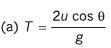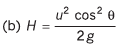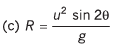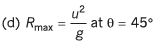Q.4. A particle is projected from ground with velocity 40√2 m/s at 45º
Find:
(a) velocity and
(b) displacement of the particle after 2 s. (g = 10 m/s2)
Ans. (a) 20√5 m/s at angle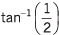with horizontal, (b) 100 m

u = 40√2 m/s, θ = 45°
As horizontal acceleration would be zero.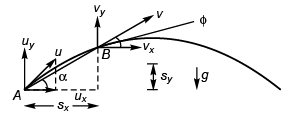vx = ux = u cos θ = 40 m/s
sx = uxt = (u cos θ) t = 80 m
A : position of particle at time = 0.
B : position of particle at time = t.
As vertical acceleration would be - g
vy = uy - gt
= u sin θ = gt
= 40 - 20
= 20 m/s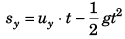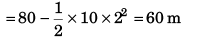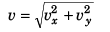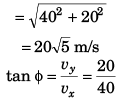i.e.,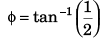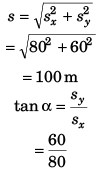i.e.,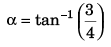Q.5. A particle is projected from ground with velocity 20√2 m/s at 45°. At what time particle is at height 15 m from ground? (g = 10 m/s2)
Ans. 1 s and 3 s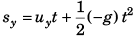or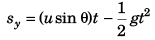or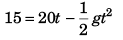or  t2 = 4t + 3 = 0
i.e., t = 1  s and 3 s

Q.6. A particle is projected from ground with velocity 40 m/s at 60° with horizontal. Find speed of particle when its velocity is making 45° with horizontal. Also find the times (s) when it happens, (g = 10 m/s2)
Ans.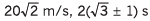See figure to the answer to question no. 4.
u = 40 m/s, θ = 60°
∴ ux = 40 cos 60° = 20 m/s
Thus, vx = ux = 20 m/s
As φ = 45°∴ yy = vx = 20 m/s
Thus,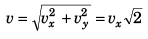= 20√2 m/s
Before reaching highest point
vy = uy + (- g) t

∴ 20 = 40 sin 60° - 10 t
or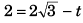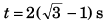After attaining highest point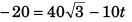i.e.,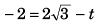or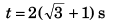Q.7. What is the average velocity of a particle projected from the ground with speed u at an angle a with the horizontal over a time interval from beginning till it strikes the ground again?
Ans. u cos α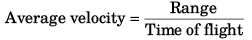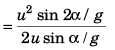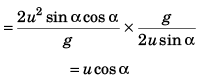Q.8. What is the change in velocity in the above question?
Ans. 2u sin α (downwards)

Change in velocity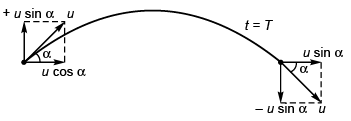= (- u sin α) - (+ u sin α)
= - 2u sin α
= 2u sin α (downward)

Q.9. Under what conditions the formulae of range, time of flight, and maximum height can be applied directly in case of a projectile motion?
Ans. Between two points lying on the same horizontal line.

Formulae for R, T and Hmax will be same if the projection point and the point where the particle lands are same and lie on a horizontal line.

Q.10. A body is projected up such that its position vector varies with time as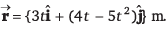Here, t is in seconds.

Find the time and x-coordinate of particle when its y-coordinate is zero.
Ans. time = 0, 0.8 s, x-coordinate = 0, 2.4 m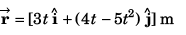y-coordinate will be zero when 4t - 5t2 = 0
i.e.,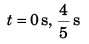t = 0 belongs to the initial point of projection of the particle.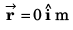i.e., x = 0 m
At t = 0.8 s,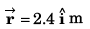i.e.,   x = 2.4 m

Q.11. A particle is projected at an angle 60° with horizontal with a speed v = 20 m/s. Taking g = 10 m/s2. Find the time after which the speed of the particle remains half of its initial speed.
Ans.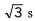vx = ux = 10 m/s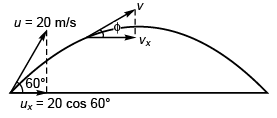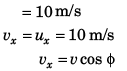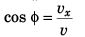= 10/v
= 10/10
[as, v = 20/2(given)]
= 1 i.e.,    φ = 0°
∴ Speed will be half of its initial value at the highest point where φ = 0°.
Thus,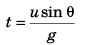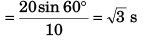Offer running on EduRev: Apply code STAYHOME200 to get INR 200 off on our premium plan EduRev Infinity!

## Physics For JEE

187 videos|516 docs|263 tests

,

,

,

,

,

,

,

,

,

,

,

,

,

,

,

,

,

,

,

,

,

,

,

,

;